Browse by Topic

# Drawing on Autopilot: Automated Plane (Geometry) Illustrations from The American Mathematical Monthly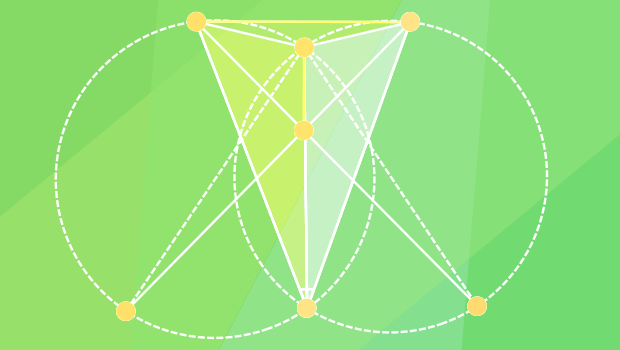Version 12 of the Wolfram Language introduces the functions GeometricScene, RandomInstance and FindGeometricConjectures for representing, drawing and reasoning about problems in plane geometry. In particular, abstract scene descriptions can be automatically supplied with coordinate values to produce diagrams satisfying the conditions of the scene. Let’s apply this functionality to some of the articles and problems about geometry appearing in the issues of The American Mathematical Monthly from February and March of 2019.

## Solving Newton’s Equation Geometrically

First consider the article “Newton Quadrilaterals, the Associated Cubic Equations, and Their Rational Solutions,” by Mowaffaq Hajja and Jonathan Sondow, appearing in the February 2019 issue.

Newton posed the following problem in his 1720 algebra textbook Universal Arithmetick: given a quadrilateral with side lengths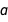,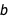,and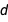inscribed in a circle of diameter, solve forgiven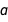,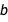and. His solution was Newton’s equation: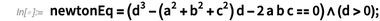✕ `newtonEq=(d^3-(a^2+b^2+c^2)d-2a b c==0)∧(d>0);`

Let’s solve Newton’s equation for the following random values of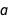,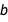and: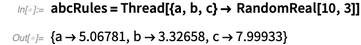✕ `abcRules=Thread[{a,b,c}-> RandomReal[10,3]]`

We could use Solve to find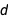directly: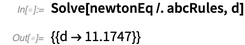✕ `Solve[newtonEq/.abcRules,d]`

We could also employ RandomInstance and GeometricScene to solve forusing the original geometric construction. First we draw the scene, only using the values of,and(the symbol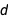appears, but is not assigned a value initially; the first argument of GeometricScene contains the list of symbolic points and, optionally, the list of symbolic quantities, each of which can be given a fixed value via a rule assignment, if desired):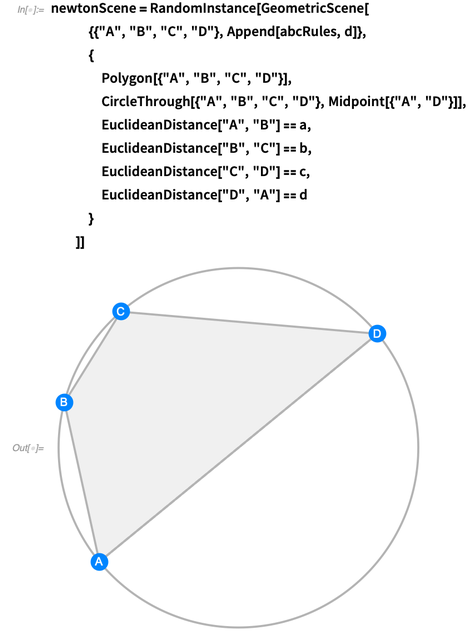✕ ```newtonScene=RandomInstance[GeometricScene[ {{"A","B","C","D"},Append[abcRules,d]}, { Polygon[{"A","B","C","D"}], CircleThrough[{"A","B","C","D"},Midpoint[{"A","D"}]], EuclideanDistance["A","B"]==a, EuclideanDistance["B","C"]==b, EuclideanDistance["C","D"]==c, EuclideanDistance["D","A"]==d } ]]```

Now we extract the value ofin this scene, and see that it equals our solution found directly: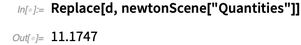✕ `Replace[d,newtonScene["Quantities"]]`

The present-day authors prove the converse of Newton’s original statement: given positive numbers,,andsatisfying Newton’s equation, there exists a quadrilateral with side lengths,,andinscribed in a circle of diameter.

We find an instance of such values for,,and: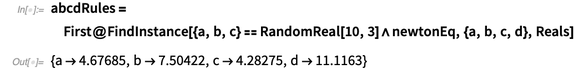✕ `abcdRules=First@FindInstance[{a,b,c}==RandomReal[10,3]∧newtonEq,{a,b,c,d},Reals]`

Indeed, we can draw the scene: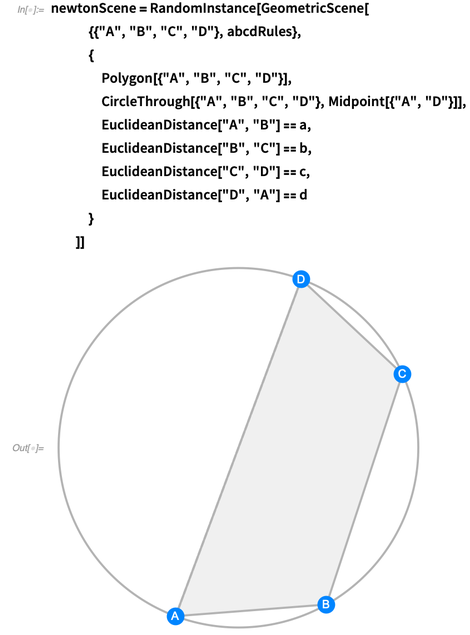✕ ```newtonScene=RandomInstance[GeometricScene[ {{"A","B","C","D"},abcdRules}, { Polygon[{"A","B","C","D"}], CircleThrough[{"A","B","C","D"},Midpoint[{"A","D"}]], EuclideanDistance["A","B"]==a, EuclideanDistance["B","C"]==b, EuclideanDistance["C","D"]==c, EuclideanDistance["D","A"]==d } ]]```

## Illustrating a Geometric Problem and Conjecturing Its Conclusion

Next we consider Problem 12092 from the February 2019 Problems and Solutions section, proposed by Michael Diao and Andrew Wu.

Let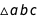be a triangle, and let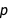be a point in the plane of the triangle satisfying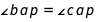. Let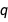andbe diametrically oppositeon the circumcircles of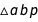and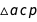, respectively. Let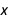be the point of concurrency of lines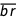and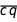. Prove that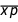and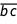are perpendicular.

Illustrate the hypotheses: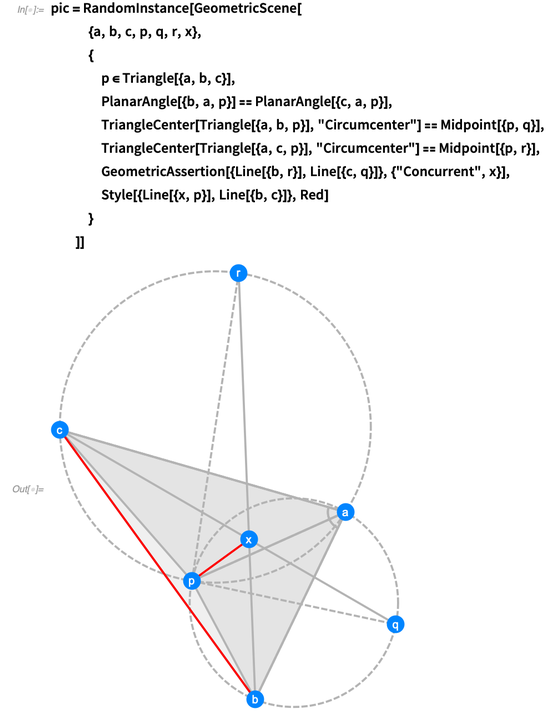✕ ```pic=RandomInstance[GeometricScene[ {a,b,c,p,q,r,x}, { p∈Triangle[{a,b,c}], PlanarAngle[{b,a,p}]==PlanarAngle[{c,a,p}], TriangleCenter[Triangle[{a,b,p}],"Circumcenter"]==Midpoint[{p,q}], TriangleCenter[Triangle[{a,c,p}],"Circumcenter"]==Midpoint[{p,r}], GeometricAssertion[{Line[{b,r}],Line[{c,q}]},{"Concurrent",x}], Style[{Line[{x,p}],Line[{b,c}]},Red] } ]]```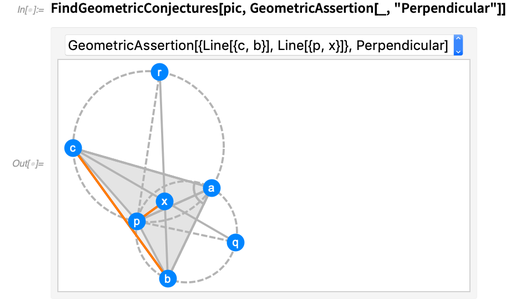✕ `FindGeometricConjectures[pic,GeometricAssertion[_,"Perpendicular"]]`

## Finding Evidence in Support of Geometric Inequalities

Finally, we consider Problem 12098 from the March 2019 Problems and Solutions section, proposed by Leonard Giugiuc and Kadir Altintas.

Suppose that the centroid of a triangle with semiperimeterand inradiuslies on its incircle. Prove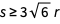, and determine conditions for equality.

Generate three separate instances of the scene: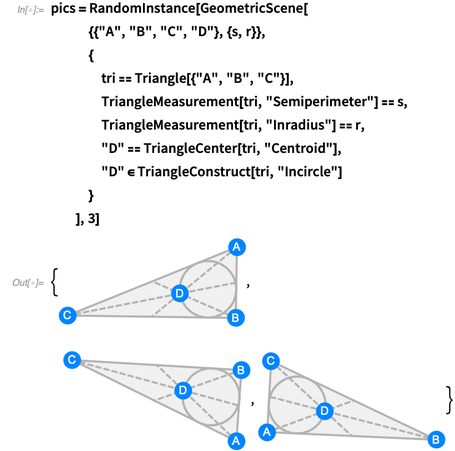✕ ```pics=RandomInstance[GeometricScene[ {{"A","B","C","D"},{s,r}}, { tri==Triangle[{"A","B","C"}], TriangleMeasurement[tri,"Semiperimeter"]==s, TriangleMeasurement[tri,"Inradius"]==r, "D"==TriangleCenter[tri,"Centroid"], "D"∈TriangleConstruct[tri,"Incircle"] } ],3]```

Verify that the inequality holds in each instance: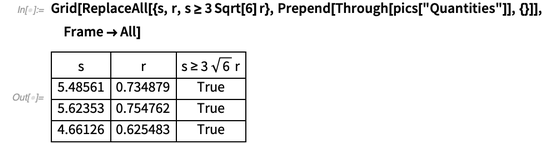✕ `Grid[ReplaceAll[{s,r,s>=3Sqrtr},Prepend[Through[pics["Quantities"]],{}]],Frame->All]`

Verify that the inequality holds in general for triangles having side lengths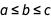, using the formulas for semiperimeter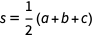, inradius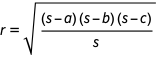and distance from incenter to centroid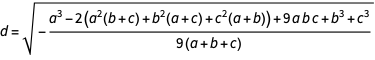: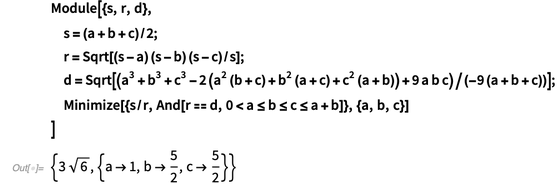✕ ```Module[{s,r,d}, s=(a+b+c)/2; r=Sqrt[(s-a)(s-b)(s-c)/s]; d=Sqrt[(a^3+b^3+c^3-2(a^2 (b+c)+b^2 (a+c)+c^2 (a+b))+9a b c)/(-9(a+b+c))]; Minimize[{s/r,And[r==d,0 ```

Since the minimum value of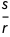, taken over all side lengths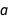,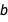andsatisfying the given constraints, is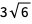(realized with side lengths,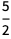and), the inequalityholds in general.

Find three instances where equality holds: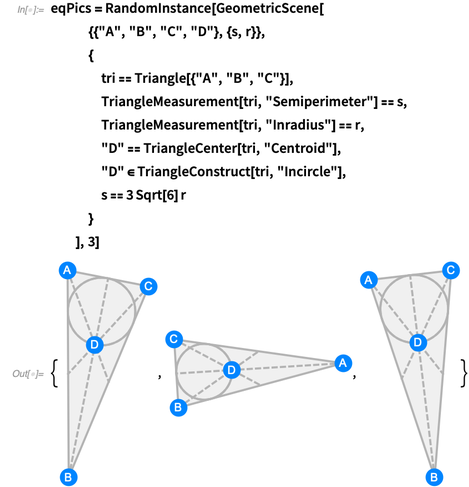✕ ```eqPics=RandomInstance[GeometricScene[ {{"A","B","C","D"},{s,r}}, { tri==Triangle[{"A","B","C"}], TriangleMeasurement[tri,"Semiperimeter"]==s, TriangleMeasurement[tri,"Inradius"]==r, "D"==TriangleCenter[tri,"Centroid"], "D"∈TriangleConstruct[tri,"Incircle"], s==3Sqrtr } ],3]```

These triangles all look suspiciously similar. To confirm our suspicions, we order the side lengths of each triangle asand then compute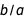and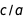: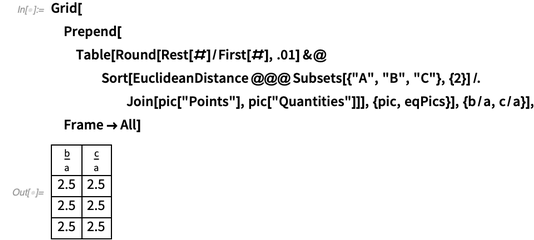✕ `Grid[Prepend[Table[Round[Rest[#]/First[#],.01]&@Sort[EuclideanDistance@@@Subsets[{"A","B","C"},{2}]/.Join[pic["Points"],pic["Quantities"]]],{pic,eqPics}],{b/a,c/a}],Frame->All]`

Indeed, all triangles seem to be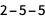isosceles triangles. To prove this holds in general, we find all possible values of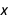and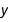for the triangle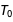having coordinates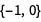,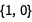and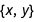to satisfy the stated properties: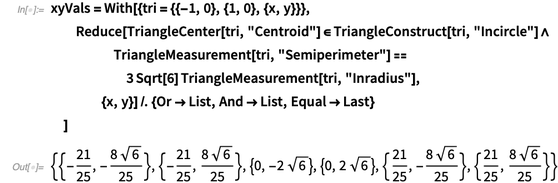✕ ```xyVals=With[{tri={{-1,0},{1,0},{x,y}}}, Reduce[TriangleCenter[tri,"Centroid"]∈TriangleConstruct[tri,"Incircle"]∧TriangleMeasurement[tri,"Semiperimeter"]==3SqrtTriangleMeasurement[tri,"Inradius"], {x,y}]/.{Or->List,And->List,Equal->Last} ]```

Visualize these triangles: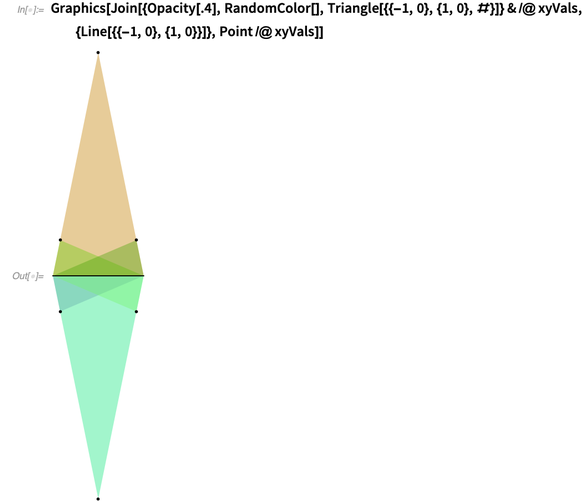✕ `Graphics[Join[{Opacity[.4],RandomColor[],Triangle[{{-1,0},{1,0},#}]}&/@xyVals,{Line[{{-1,0},{1,0}}]},Point/@xyVals]]`

Verify that the triangles satisfying the equality are all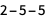isosceles triangles, proving our claim in general: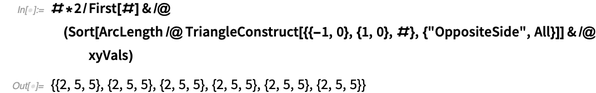✕ `#*2/First[#]&/@(Sort[ArcLength/@TriangleConstruct[{{-1,0},{1,0},#},{"OppositeSide",All}]]&/@xyVals)`

Hence we have demonstrated that the inequality holds in general, with equality for a single class of similar triangles. Isosceleasy-peasy!

 Building on three decades of development, Mathematica excels across all areas of technical computing—including machine learning, image processing, geometry, visualizations, and much more. Buy now!